# Civil Engineering - Hydraulics

### Exercise :: Hydraulics - Section 1

36.

When a body is totally or partially immersed in a fluid, it is buoyed up by a force equal to

 A. weight of the body B. weight of the fluid displaced by the body C. weight of the body and fluild displaced by the body D. difference of weights of the fluid displaced and that of the body E. none of these.

Explanation:

No answer description available for this question. Let us discuss.

37.

If D is the diameter of a pipe of length L and f is the coefficient of friction of pipe then diameter of the nozzle d is

 A.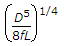B.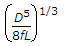C.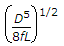D. none of these.

Explanation:

No answer description available for this question. Let us discuss.

38.

If the total head of the nozzle of a pipe is 37.5 m and discharge is 1 cumec, the power generated is

 A. 400 H.P. B. 450 H.P. C. 500 H.P. D. 550 H.P.

Explanation:

No answer description available for this question. Let us discuss.

39.

The phenomenon occuring in an open channel when a rapidly flowing stream abruptly changes to a slowly flowing stream causing a distinct rise of liquid surface, is

 A. water hammer B. hydraulic jump C. critical discharge D. none of these.

Explanation:

No answer description available for this question. Let us discuss.

40.

In a circular pipe of length L and diameter d, a viscous liquid is flowing with a velocity v. The loss in head, is

 A.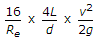B.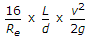C.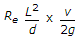D.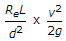E. none of these.